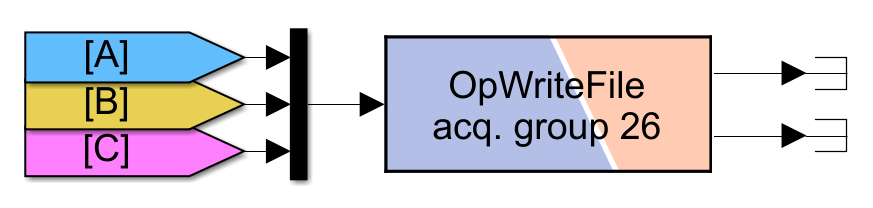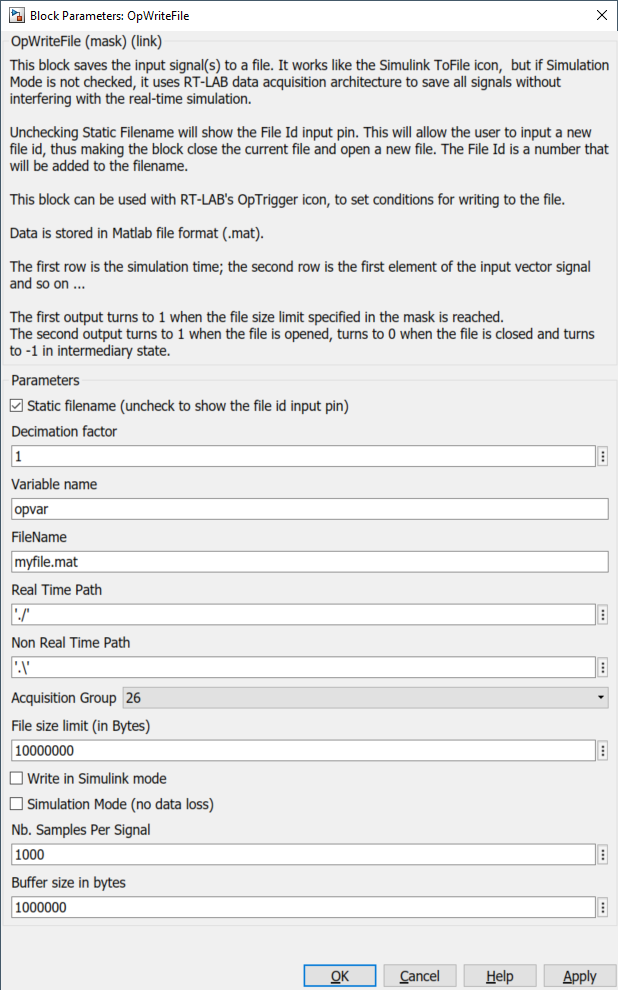# Knowledge Base

### Welcome to OPAL-RT’s Knowledge Base

OPAL-RT offers a repository of support information for optimal use of its technology.

Please note that OPAL-RT knowledge base is not fully optimized for mobile platforms.

For optimal experience, use a desktop computer.

Reference Number: AA-00872 // Views: 4260 // Created: 2013-08-08 05:00:00 // Last Updated: 2022-05-27 20:58:58
HowTo
How to Use and Configure the OpWriteFile Block

This article presents the basic concepts of the OpWriteFile block, introduces the key parameters and discusses some extra considerations.

The OpWriteFile block is part of the RT-LAB > DataLogging Simulink library.

## 1) Basic Concept

The goal of the OpWriteFile block is to allow the user to record signals. The main advantages are:

• Saves the results into a .mat file. Useful for post-processing purposes.
• Records directly on the target. In other words, it does not use the TCP/IP link to record data (thus avoid potential data loss).
• An OpWriteFile block can be triggered to start the acquisition (see section 3) and can record a new file once the first recording is complete.

The Simulink signals that need to be logged to the MAT-File should be connected to the OpWriteFile block input using a Mux block.

For example:Once the logging is finished, users get a MAT-File with a 2D array, which is composed of:

• 1st row = Time (Automatically added)
• n additional rows, where n = the number of signals fed to the OpWriteFile block input
• Each column corresponds to one sample, as in the figure belowNotes

• Once the logging is completed or the model is reset, the file is transferred back to the localhost computer (i.e. the Windows computer running RT-LAB) in the OpREDHAWKtarget folder of the corresponding subsystem.
• For instance, if the model is named rtdemo1 and the OpWriteFile block is in the 'sm_computation' subsystem, then the file is transferred back to this path:
<Project directory>\models\rtdemo1\rtdemo1_sm_computation\OpREDHAWKtarget\ <name of file>.mat
•  CAREFUL! If the same simulation is run again, the file created during the previous simulation will be overwritten. The file needs to be copied elsewhere before starting another simulation.
• If the model is recompiled, the OpREDHAWKtarget folder gets overwritten too.

## 2) Mask of OpWriteFile Block

Here is the mask of the OpWriteFile block:The key parameters are: Buffer Size, Nb. Samples Per Signal, Decimation Factor and File Size Limit.

These parameters define how the acquisition of data is performed. If these parameters are well-chosen, the acquisition will work fine. Otherwise, some data may be lost or the file may be empty. See below for properly setting those parameters.

### 2.1) Buffer Size in Bytes & Nb. Samples

The recorded signals are not directly written in the .mat file, the samples of the logged signals are temporarily logged into a bank of memory call buffer first.

While the simulation is running, the signals are copied into the buffer until the number of copied samples reaches Nb. samples.

Then the buffer's data is transferred to the MAT-File. In parallel, a second buffer starts recording.The buffer must be able to contain all the samples at each iteration. Therefore, buffer size must be greater than the results of the below equations. See the following Note for details:

Buffer size (bytes) >= (Number of logged signals + 1) x (Nb. samples) x 8

Note:

• The Time signal is automatically added as in the first row; thus, the +1 for the number of logged signals.
• A double (Simulink signal type is double by default) is composed of 64 bits or 8 bytes; thus, the x8 at the end to have the size in bytes

### 2.2) Decimation Factor

The Decimation factor specifies the sampling factor. If set to 5, a sample for each signal is logged every 5 model time steps.

Examples:### 2.3) File Size Limit

The File Size Limit parameter is to ensure that the transfer time is not too long (a file can reach a size of several Gb in a few minutes).

The file size limit can be calculated with:

File size (bytes) = 1.5*(Number of logged signals +  1) x 8 x (Logging duration(s)) / (Decimation factor x Sample time(s))

Example

• Number of logged signals = 10
• Logging duration = 60 s
• Decimation factor = 1 (all samples are logged)
• Sample time = 50 µs (50e-6 s)

File size = 158400000 bytes = 158.4 Mb

Notes:

• The factor of 1.5 at the beginning is because there is an extra header space that must be taken into account when calculating the File size for the OpWriteFile block. This header varies according to the number of signals and other factors. Therefore, 1.5 is a rule of thumb to make sure the file size is sufficient, but it can be modified depending on the use case.
• A double (Simulink signal type is double by default) is composed of 64 bits or 8 bytes; thus, the x8 to have the size in bytes.

### 2.4) Parameters FileName and Variable Name

The parameter FileName specifies the name of the MAT-File containing the acquired data. This file is automatically downloaded to the host PC once the file is closed (when the model is reset).

Parameter Variable name specifies the name of the array located in the MAT-File that contains all the logged data (i.e. the name in the Matlab workspace).

### 3.1) Writing Time Interval

Under the hood, there each OpWriteFile block actually has two identical buffers. When the first one is full, it gets transferred to the mat file and the second starts filling up. The user must ensure that the time interval between 2 write operations in the mat file is big enough (i.e. from the buffer to the mat file) so that the second buffer is not full before the first one finished transferring the data to the mat file. Furthermore, reading or writing in a file is a time-consuming task, so it is better not to do it too often!

A time interval between hundreds of milliseconds and seconds is acceptable.

The writing time interval can be calculated from the Decimation Factor and the Nb. Samples, as follows:

Writing time interval (s) = Nb. Samples * Sample time (s) * Decimation factor

Example 1:

• Sample time = 100 µs
• Decimation factor = 1
• Nb. Samples = 10

Writing time interval = 10 * 100e-6 * 1 = 1 ms (too fast!)

Example 2:

• Sample time = 1 ms
• Decimation factor = 2
• Nb. Samples = 500

Writing time interval = 500* 1e-3 * 2 = 1 s (good!)

### 3.2) Deciding when the OpWriteFile Starts to Record

By default, the OpWriteFile starts to record from the beginning of the simulation. To control the beginning of the recording, one can use the OpTrigger. Please consult the RT-LAB documentation for more information.

### 3.3) Retrieving the File While the Simulation Is Running

By default, the file will only be transferred back when the simulation is reset. However, it is possible to retrieve the file while the simulation is running (settings in RT-LAB).